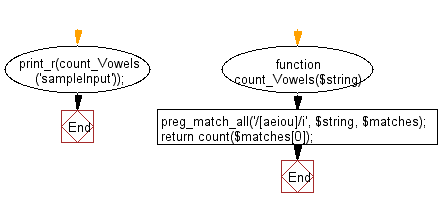﻿ PHP Exercise: Count number of vowels in a given string - w3resource

# PHP Exercises: Count number of vowels in a given string

## PHP: Exercise-96 with Solution

Write a PHP program to count number of vowels in a given string.

Note: Use a regular expression to count the number of vowels (A, E, I, O, U) in a string.

Sample Solution: -

PHP Code:

``````<?php
//Licence: https://bit.ly/2CFA5XY

function count_Vowels(\$string)
{
preg_match_all('/[aeiou]/i', \$string, \$matches);
return count(\$matches);
}
print_r(count_Vowels('sampleInput'));

?>
```
```

Sample Output:

```4
```

Flowchart:PHP Code Editor:

Have another way to solve this solution? Contribute your code (and comments) through Disqus.

What is the difficulty level of this exercise?

Test your Programming skills with w3resource's quiz.

﻿

## PHP: Tips of the Day

Mutates the original array to filter out the values specified

Example:

```<?php
function tips_pull(&\$items, ...\$params)
{
\$items = array_values(array_diff(\$items, \$params));
return \$items;
}

\$items = ['x', 'y', 'z', 'x', 'y', 'z'];
print_r(tips_pull(\$items, 'y', 'z'));
?>
```

Output:

```Array
(
 => x
 => x
)
```